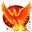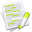#wx.lib.floatcanvas.FCObjects.Polygon¶

Draws a polygon

Points is a list of 2-tuples, or a NX2 NumPy array of point coordinates. so that Points[N] is the x-coordinate of point N and Points[N] is the y-coordinate or Points[N,0] is the x-coordinate of point N and Points[N,1] is the y-coordinate for arrays.

##Class Hierarchy¶Inheritance diagram for class Polygon:

##Methods Summary¶

 __init__ Default class constructor.

##Class API¶

class Polygon(PointsObjectMixin, LineAndFillMixin, DrawObject)

Draws a polygon

Points is a list of 2-tuples, or a NX2 NumPy array of point coordinates. so that Points[N] is the x-coordinate of point N and Points[N] is the y-coordinate or Points[N,0] is the x-coordinate of point N and Points[N,1] is the y-coordinate for arrays.

### Methods¶

__init__(self, Points, LineColor = "Black", LineStyle = "Solid", LineWidth = 1, FillColor = None, FillStyle = "Solid", InForeground = False)

Default class constructor.

Parameters
• Points – start point, takes a 2-tuple, or a (2,) NumPy array of point coordinates

• LineColor – see SetColor

• LineStyle – see SetLineStyle

• LineWidth – see SetLineWidth

• FillColor – see SetColor

• FillStyle – see SetFillStyle

• InForeground (boolean) – should object be in foreground